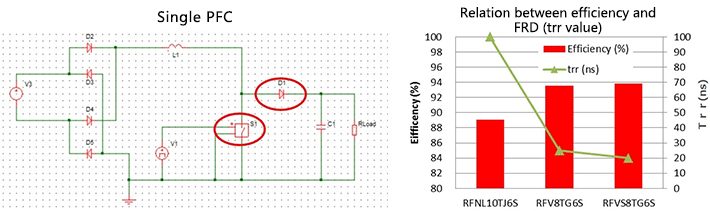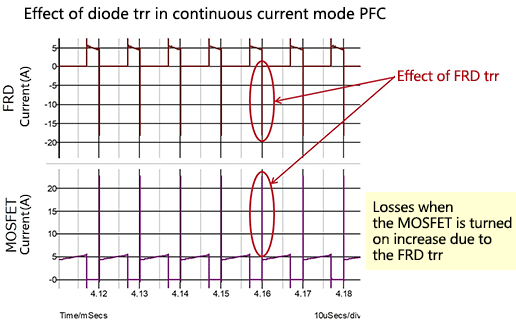Technical Information Site of Power Supply Design

2018.09.06 Si Power Device

# Continuous Current Mode PFC：Examples of Efficiency Improvement Using Diode

Application Examples that Exploit Feature

In succession to the boundary current mode PFC example of the previous article, this time we present an example of efficiency improvement in continuous current mode PFC through differences in diode characteristics.

Example of Efficiency Improvement in a Continuous Current Mode PFC Circuit Using Diodes

This is a simplified PFC circuit example that was used in the previous PFC explanation. In the diode and MOSFET combination that is the main part of the PFC output stage, we consider the influence of the diode characteristics on the efficiency. The diode used is a FRD (fast recovery diode); measured results for the efficiency using diodes with three different characteristics are shown.The graph on the right superposes the FRD trr (reverse recovery time) versus circuit efficiency for each of the FRDs. As can be seen from the graph, the highest efficiency results when using the FRD with the shortest trr. Below, the main characteristics and measured efficiency for each FRD are presented.

FRD IF (A) VF (V)
Typ. @IF max
trr (ns)
Typ. @IF max, VR=400V
Efficiency (%)
RFNL10TJ6S 10 1.1 100 (dIF/dt=-100A) 89.10
RFV8TG6S 8 2.3 25 (dIF/dt=-200A) 93.59
RFVS8TG6S 8 2.5 20 (dIF/dt=-200A) 93.87

Circuit conditions: Continuous current mode, Po=300 W, fsw=200 kHz, Vin=115 Vrms, Vo=390 V

RFNL10TJ6S and RFV08TJ6S are FRDs that were used in boundary current mode PFC loss simulations in the previous article; the RFNL10TJ6S, with the lowest VF, was the FRD for which the highest efficiency in boundary current mode PFC was recorded. Conversely, the RFV08TJ6S has a higher VF than the RFNL10TJ6S, and so in boundary current mode PFC resulted in lower efficiency.

However, the VF has almost no influence on efficiency in continuous current mode PFC, whereas the effect of the trr dominates. The reason for the reduced efficiency when the trr is slow can be inferred from the following waveforms.In the FRD waveforms, the FRD turns on and a forward current IF of about 5 A flows, and when the device is then turned off a reverse current of about 18 A flows. This IR flows during the trr period, and so in continuous current mode PFC, this current affects the MOSFET switching. As the waveforms indicate, when the MOSFET is turned on a large spike-shape current flows, and this becomes a loss, lowering the efficiency of the overall circuit. For details of FRD trr values, please refer to this page.

In conclusion, in continuous current mode PFC the efficiency is higher for lower diode trr values. VF has essentially no influence on the efficiency.

#### Key Points:

・In continuous current mode (CCM) PFC, the diode trr has a major effect on losses, whereas the effect of the diode VF is small.

・In PFC under continuous current mode control, by selecting a diode with a low trr, circuit efficiency can be improved.